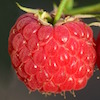Equations and Formulae - Stage 3What's it Worth?

Age 11 to 16 Challenge Level:

There are lots of different methods to find out what the shapes are worth - how many can you find?Temperature

Age 11 to 14 Challenge Level:

Is there a temperature at which Celsius and Fahrenheit readings are the same?Age 11 to 14 Challenge Level:

Think of a number and follow my instructions. Tell me your answer, and I'll tell you what you started with! Can you explain how I know?Multiplication Mistake

Age 11 to 14 Short Challenge Level:

Jane accidentally multiplied by 54 instead of 45, and her answer was 198 too big. What number did she multiply 54 by?Fruity Totals

Age 7 to 16 Challenge Level:

In this interactivity each fruit has a hidden value. Can you deduce what each one is worth?